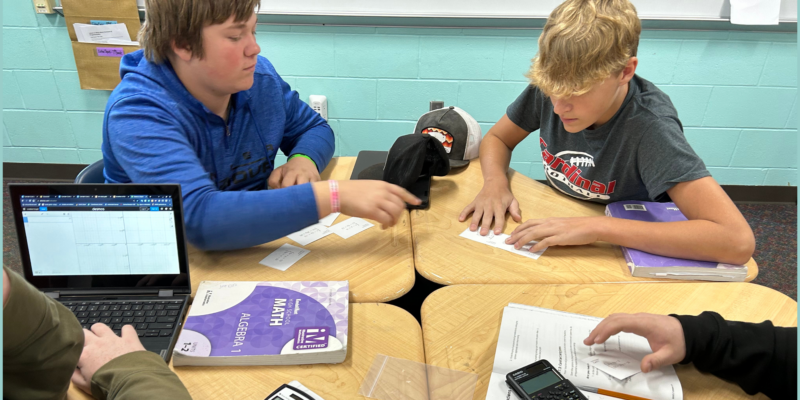# Algebra I Students Study Systems of Linear EquationsAlgebra 1 classes were working on an activity for their lesson over systems of linear Equations and their solutions in October.

In earlier activities, students gained some insights on the structure of equations in systems that have infinitely many solutions and those that have no solutions. In this activity, they apply those insights to sort systems of equations based on the number of solutions (one solution, many solutions, or no solutions).  To effectively make use of the structure of the systems, students need to attend closely to all parts of each equation—the signs, variables, coefficients and constants—and to rearrange equations with care.

As students discuss their thinking in groups,. they are solving individual systems to analyze the features of the equations and see if they could reason about the solutions or gain information about the graphs that way.  Students could then check their answer by using Technology and our Desmos Graphing Tool.